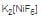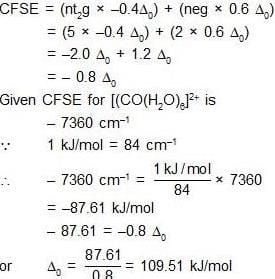Coordination - Organometallics - Bioinorganic

# Coordination - Organometallics - Bioinorganic

Test Description

## 60 Questions MCQ Test Mock Test Series for IIT JAM Chemistry | Coordination - Organometallics - Bioinorganic

Coordination - Organometallics - Bioinorganic for Chemistry 2022 is part of Mock Test Series for IIT JAM Chemistry preparation. The Coordination - Organometallics - Bioinorganic questions and answers have been prepared according to the Chemistry exam syllabus.The Coordination - Organometallics - Bioinorganic MCQs are made for Chemistry 2022 Exam. Find important definitions, questions, notes, meanings, examples, exercises, MCQs and online tests for Coordination - Organometallics - Bioinorganic below.
Solutions of Coordination - Organometallics - Bioinorganic questions in English are available as part of our Mock Test Series for IIT JAM Chemistry for Chemistry & Coordination - Organometallics - Bioinorganic solutions in Hindi for Mock Test Series for IIT JAM Chemistry course. Download more important topics, notes, lectures and mock test series for Chemistry Exam by signing up for free. Attempt Coordination - Organometallics - Bioinorganic | 60 questions in 180 minutes | Mock test for Chemistry preparation | Free important questions MCQ to study Mock Test Series for IIT JAM Chemistry for Chemistry Exam | Download free PDF with solutions
 1 Crore+ students have signed up on EduRev. Have you?
Coordination - Organometallics - Bioinorganic - Question 1

### Which of the following least covalent or least acidic?

Coordination - Organometallics - Bioinorganic - Question 2

### Spin motion of which of the following gives magnetic moment?         I. Electron II. Proton III. Neutron

Coordination - Organometallics - Bioinorganic - Question 3

### Consider the coordination compound Na2[Pt(CN)4]. The Lewis acid is?

Coordination - Organometallics - Bioinorganic - Question 4

Using CFT, identify the following complex ions that shows same Ueff (spin only) value?
I. [CoF6]3-                              II. [IrCl6]3-                       III. [Fe(H2O)6]2+

Coordination - Organometallics - Bioinorganic - Question 5

The metal ion of an enzyme involved in the hydration of CO2 is:

Coordination - Organometallics - Bioinorganic - Question 6

At pH 7.2 and 10 Torr oxygen partial pressure, the extent of O2 binding is:

Coordination - Organometallics - Bioinorganic - Question 7

For the Metalloprotein Hemerythrin, the statement that is not true is:

Coordination - Organometallics - Bioinorganic - Question 8

Os5(CO)16 adopts the following structure:

Coordination - Organometallics - Bioinorganic - Question 9

What would be the oxidation of Mn in CpMn(NMe2)(OEt)(CPh2) ?

Coordination - Organometallics - Bioinorganic - Question 10

Consider the compound [M(η6-C6H6)(CS)2]2. Metal ‘M’ can be

Coordination - Organometallics - Bioinorganic - Question 11

Ground state term for V3+ ion is:

Coordination - Organometallics - Bioinorganic - Question 12

The compound which shows MLCT is:

Coordination - Organometallics - Bioinorganic - Question 13

Type of isomerism which exists between [Pd(C6H5)2(SCN)2] and [Pd(C6H5)2(NCS)2] is:

Coordination - Organometallics - Bioinorganic - Question 14

Nitrosyl ligands bind to d-metal atoms in linear and bent fashion and behaves, respectively as

Coordination - Organometallics - Bioinorganic - Question 15

For tetrahedral complexes, which always exhibit high spin states, the maximum CFSE is:

Coordination - Organometallics - Bioinorganic - Question 16

Select 18especies among following:

Coordination - Organometallics - Bioinorganic - Question 17

Electron transfer from [Fe(H2O)6]2+ to [Fe(H2O)6]3+ is likely to occur viavia

Coordination - Organometallics - Bioinorganic - Question 18

B3H7[Fe(CO)3]2 adopts the following structure:

Coordination - Organometallics - Bioinorganic - Question 19

Which of the following Metallocene has 1.73 BM value of magnetic moment?

Coordination - Organometallics - Bioinorganic - Question 20

[CpMoCl2]2 obeys the 18 electron rule. The correct structure of this compound is:(atomic number of Mo = 42)

Coordination - Organometallics - Bioinorganic - Question 21

Correct combination of number and size of rings present in a metal ion-porphine complex (including metal ion bearing chelate rings) is:

Coordination - Organometallics - Bioinorganic - Question 22

The correct set of the biologically essential elements is:

Coordination - Organometallics - Bioinorganic - Question 23

Haemoglobin is an oxygen carrying protein. The correct statement about oxy-haemoglobin is that:

Coordination - Organometallics - Bioinorganic - Question 24

Which of the following metal based Nitrogenase enzymes are known?

Coordination - Organometallics - Bioinorganic - Question 25

Which of the following is also known as Photo-synthetic protein?

Coordination - Organometallics - Bioinorganic - Question 26

For mono-ionic complex [UO2(NO3)3]-, the correct coordination number and geometry resp are

Coordination - Organometallics - Bioinorganic - Question 27

Chelate effect is

Coordination - Organometallics - Bioinorganic - Question 28

The complex that shows orbital contribution to the magnetic moment, is

Coordination - Organometallics - Bioinorganic - Question 29

Correct spinal structure of Co3O4 is:

Coordination - Organometallics - Bioinorganic - Question 30

The g values for Ce3+ (4f1 ) and Pr3+ (4f2 ) are, respectively

*Multiple options can be correct
Coordination - Organometallics - Bioinorganic - Question 31

Select the correct statement:

*Multiple options can be correct
Coordination - Organometallics - Bioinorganic - Question 32

Q.32    Correct statement(s) for metal alkene complexes is/are:

*Multiple options can be correct
Coordination - Organometallics - Bioinorganic - Question 33

Choose correct statement/s:

*Multiple options can be correct
Coordination - Organometallics - Bioinorganic - Question 34

Which of the following statement(s) is/are correct about the structures of Fe(CO)5.

*Multiple options can be correct
Coordination - Organometallics - Bioinorganic - Question 35

The transition metal monoxide that shows metallic conductivity is?

*Multiple options can be correct
Coordination - Organometallics - Bioinorganic - Question 36

Based on the behavior of the Metalloenzymes, consider the following statements:

*Multiple options can be correct
Coordination - Organometallics - Bioinorganic - Question 37

Which of the following is true about Porphyrin?

*Multiple options can be correct
Coordination - Organometallics - Bioinorganic - Question 38

Among the following complexes, optical activity is possible in

*Multiple options can be correct
Coordination - Organometallics - Bioinorganic - Question 39

Among the complexes K4[Cr(CN)6] (A), K4[Fe(CN)6] (B), K3[Co(CN)6] (C), K4[Mn(CN)6] (D), Jahn-Teller distortion is shown by?

*Multiple options can be correct
Coordination - Organometallics - Bioinorganic - Question 40

Which of the following complex(s) has/have only two stereoisomers:

*Answer can only contain numeric values
Coordination - Organometallics - Bioinorganic - Question 41

The maximum number of atoms in one plane in Zeise salt are………..:?

*Answer can only contain numeric values
Coordination - Organometallics - Bioinorganic - Question 42

What is the spin multiplicity of Fischer carbene?

*Answer can only contain numeric values
Coordination - Organometallics - Bioinorganic - Question 43

Number of unpaired electrons in K2[NiF6] is?

Detailed Solution for Coordination - Organometallics - Bioinorganic - Question 43

The atomic number of nickel is 28. Thus, it has 8 electrons in 3d orbital. Here, it is in +2 oxidation state which means its two electrons of its electron from 4s orbital have been removed and the 8 electrons of 3d orbital remain untouched.

Now, F- is a weak field ligand and hence does not pair the electrons in 3d orbital. This means there are 2 unpaired electrons left in 3d orbital. Hence,has 2 unpaired electrons.

*Answer can only contain numeric values
Coordination - Organometallics - Bioinorganic - Question 44

In a homogeneous catalysis reaction, 1 mole of substrate and 1 micromole of catalyst produce 1 millimole of product in 10 sec. Find turn over frequency.

*Answer can only contain numeric values
Coordination - Organometallics - Bioinorganic - Question 45

What is the coordination number of Ce in Ceric ammonium nitrate?

*Answer can only contain numeric values
Coordination - Organometallics - Bioinorganic - Question 46

The total number of possible isomers for [Co(NH3)4Cl(NO2)]+?

*Answer can only contain numeric values
Coordination - Organometallics - Bioinorganic - Question 47

Calculate the number of M-M bond in Co4(CO)12.?

*Answer can only contain numeric values
Coordination - Organometallics - Bioinorganic - Question 48

Find the value of expression (x ÷ y) for following complexes:
(A) Co2(CO)x
(B) Fe(CO)2(NO)y

*Answer can only contain numeric values
Coordination - Organometallics - Bioinorganic - Question 49

The compound [CpMn(CO)x]2 has Mn=Mn bond. What is ‘x’?

*Answer can only contain numeric values
Coordination - Organometallics - Bioinorganic - Question 50

The number of unpaired electron (s) present in the species [Fe(H2O)5(NO)]2+ which is formed during brown ring test is:

*Answer can only contain numeric values
Coordination - Organometallics - Bioinorganic - Question 51

What is the total spin of Schrock Carbene?

Detailed Solution for Coordination - Organometallics - Bioinorganic - Question 51

Schrock Carbene is Triplet
2s + 1 = 3
2s = 2
s = 1

*Answer can only contain numeric values
Coordination - Organometallics - Bioinorganic - Question 52

How many metal-metal per metal bonds in [CpMo(CO)2]2 ?

*Answer can only contain numeric values
Coordination - Organometallics - Bioinorganic - Question 53

Consider the compound: [(CO)3NiCo(CO)3]X What is ‘X’?

*Answer can only contain numeric values
Coordination - Organometallics - Bioinorganic - Question 54

The hapticity of cycloheptatriene (C7H8), in Mo(C7H8)(CO)3 is:

*Answer can only contain numeric values
Coordination - Organometallics - Bioinorganic - Question 55

The number of metal-metal bonds present in Ir4(CO)12 are:

*Answer can only contain numeric values
Coordination - Organometallics - Bioinorganic - Question 56

In bis(dimethylglyoximato) nickel(II), the sum of the number of Ni—N, Ni—O and intra-molecular hydrogen bond(s) is_______:

*Answer can only contain numeric values
Coordination - Organometallics - Bioinorganic - Question 57

2.78 g of FeSO4.xH2O on treatment with excess of BaCl2 solution gives 2.33 g of white precipitate of BaSO4. What is value of x? (Ba = 137, S = 32, Fe = 56, O = 16)

*Answer can only contain numeric values
Coordination - Organometallics - Bioinorganic - Question 58

Given that the CFSE for [Co(H2O)6]2+ is 7360 cm-1, the calculated value of ∆o in KJmol-1 is?(rounded up to one decimal places)

Detailed Solution for Coordination - Organometallics - Bioinorganic - Question 58*Answer can only contain numeric values
Coordination - Organometallics - Bioinorganic - Question 59

Magnetic moment of [RhF6]3– in B.M. is

*Answer can only contain numeric values
Coordination - Organometallics - Bioinorganic - Question 60

Assume the complex [Pd(PPh3)2(SCN)2] is diamagnetic. Give the number of possible isomers.

## Mock Test Series for IIT JAM Chemistry

2 docs|25 tests
 Use Code STAYHOME200 and get INR 200 additional OFF Use Coupon Code
Information about Coordination - Organometallics - Bioinorganic Page
In this test you can find the Exam questions for Coordination - Organometallics - Bioinorganic solved & explained in the simplest way possible. Besides giving Questions and answers for Coordination - Organometallics - Bioinorganic , EduRev gives you an ample number of Online tests for practice

2 docs|25 tests